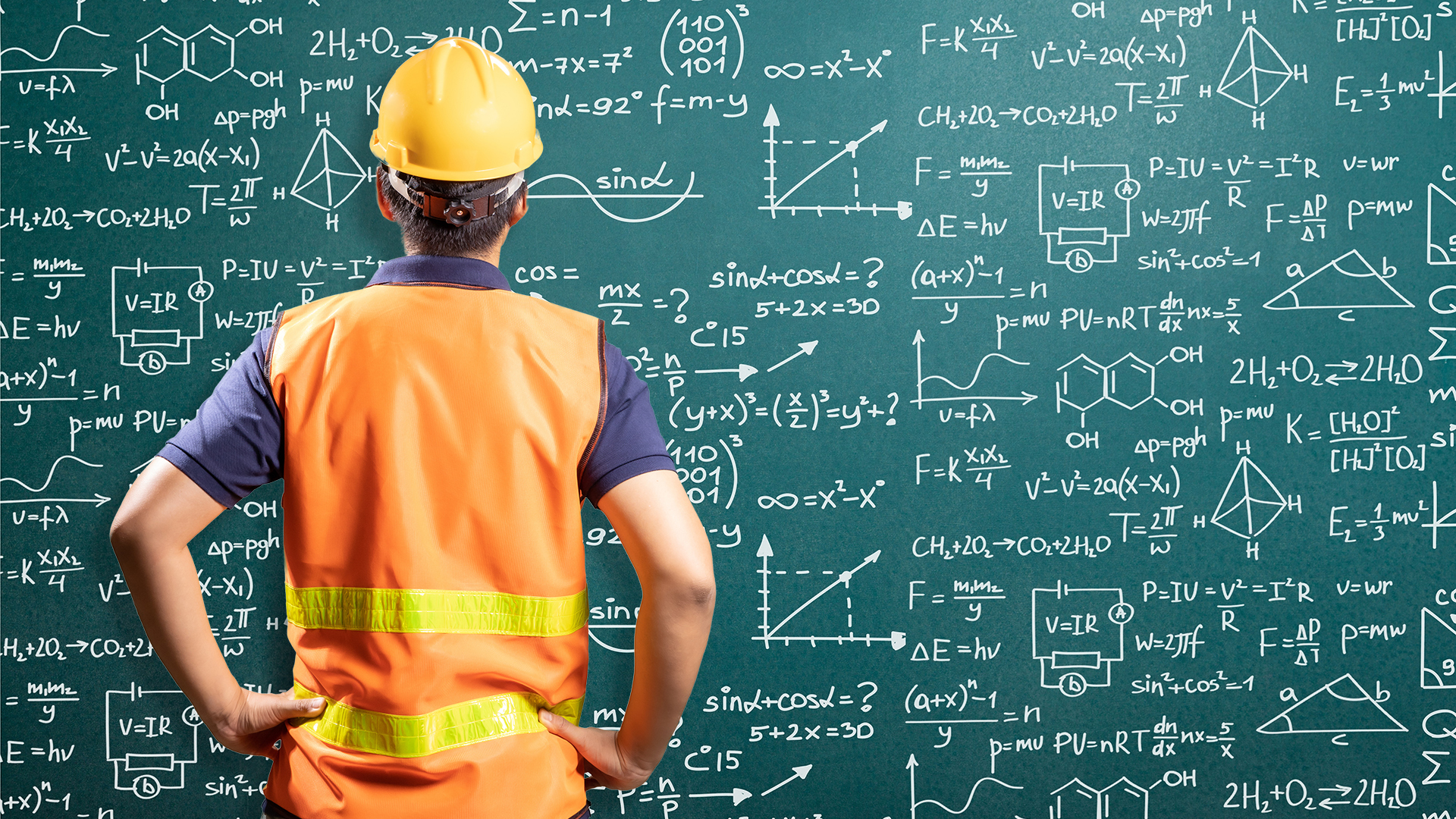# 170-02 - Industrial Math: Measurements and Calculations## Learning objectives

• Describe the use of conversion tables
• Use equations to solve problems using length, height, flow, and temperature conversions
• Solve mathematical problems using each of the following: area formulas, volumetric formulas, velocity formulas, flow formulas, density and specific gravity
• Explain how to interpret circle, bar, and line graphs
• Explain how to read and interpret charts and tables

## Course overview

When you complete this lesson, you will be able to demonstrate how to use equations to solve industrial problems involving length, height, flow and temperature conversions and calculations. You will be able to explain how to read and interpret formulas, graphs, charts, and tables. You will also be able to apply several mathematical formulas to commonly used industrial calculations.
• 50 minutes
• Format: Online Interactive
• English